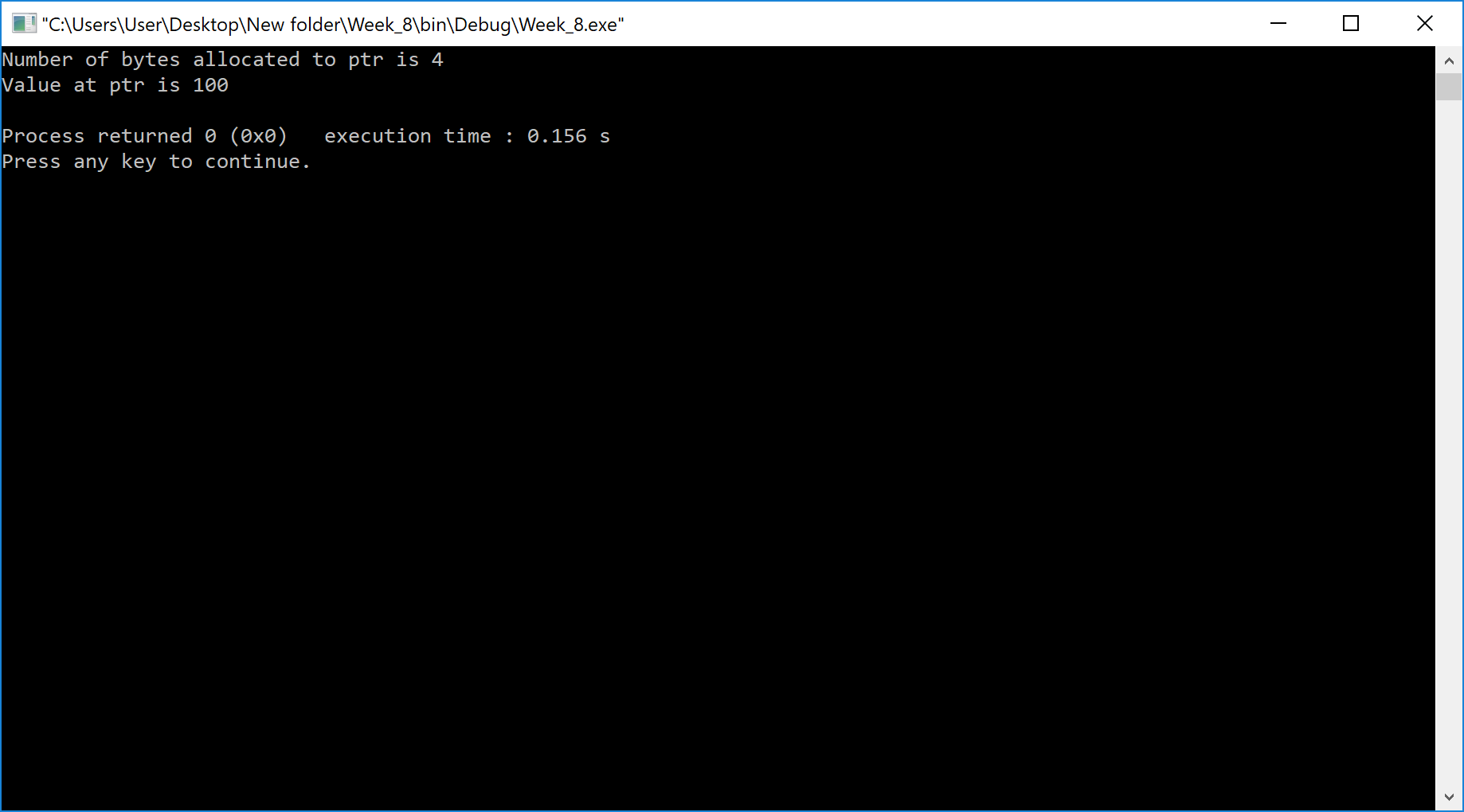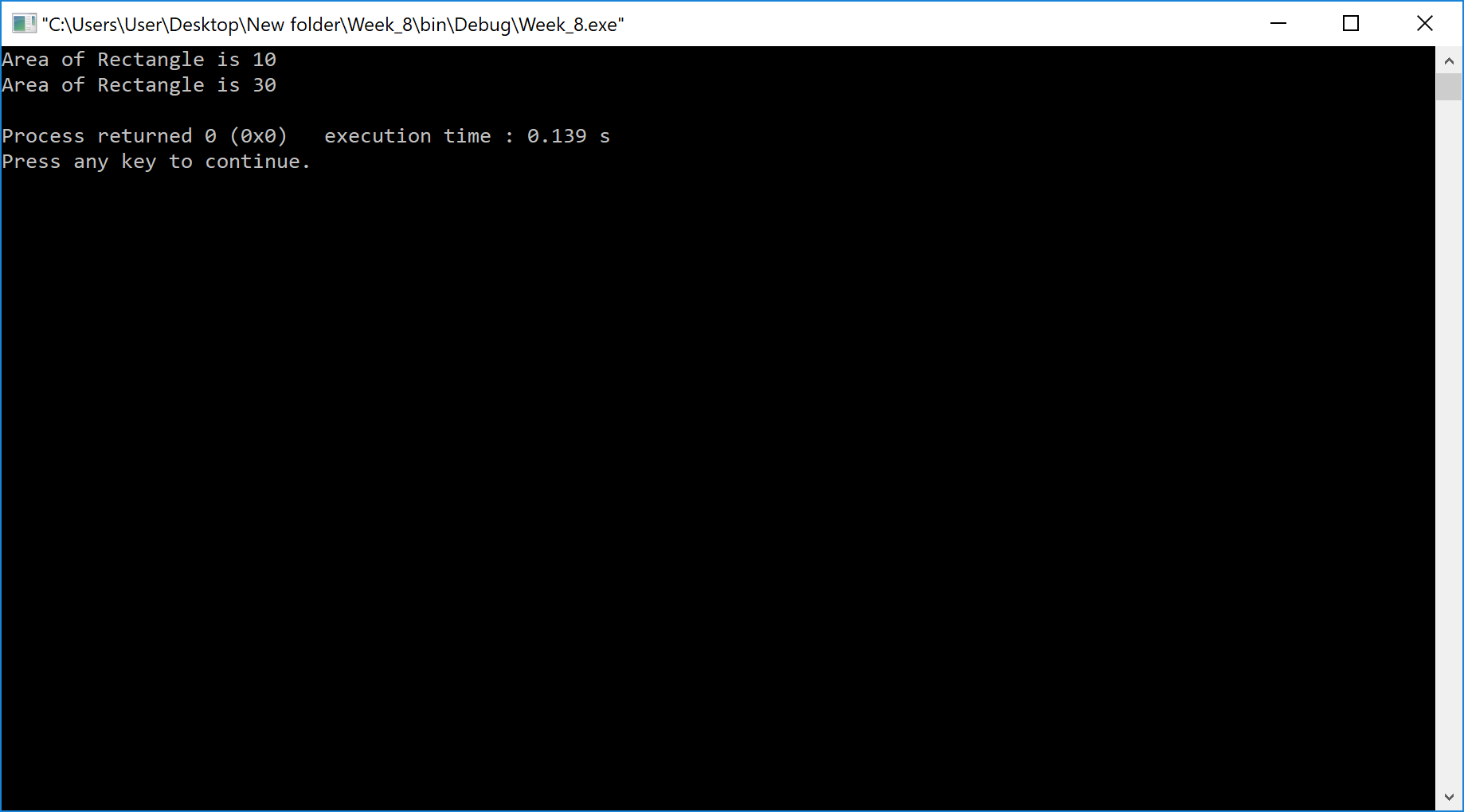The perfect place for easy learning...

×

# Aim

Write a C++ program to allocate memory using new operator.

# Solution

The dynamic memory allocation is performed at the time of program execution. In C++ programming language, we use the new operator to allocate the memory dynamically.

# ⇢ new with primitive data type variable

The memory allocation to the variables of primitive data type can be performed dynamically using new operator. Let's look at the following example program.

Example
``````#include <iostream>
using namespace std;

int main()
{
int *ptr;

ptr = new int;  // Dynamic memory allocation

cout<< "Number of bytes allocated to ptr is " << sizeof(ptr) << endl;
*ptr = 100;
cout << "Value at ptr is " << *ptr << endl;

return 0;
}
``````

# Result# ⇢ new with user-defined data type variable (Objects)

The memory allocation to the objects of a class can be performed dynamically using new operator. Let's look at the following example program.

Example
``````#include <iostream>

using namespace std;

class Rectangle{
int length, width;
public:
Rectangle(){
length = 2;
width = 5;
}
Rectangle(int l, int w){
length = l;
width = w;
}
void area(){
cout<< "Area of Rectangle is " << length * width << endl;
}
};

int main()
{
Rectangle *obj_1, *obj_2;

obj_1 = new Rectangle();  // Dynamic memory allocation
obj_2 = new Rectangle(3, 10);  // Dynamic memory allocation

obj_1->area();
obj_2->area();

return 0;
}
``````

# Result